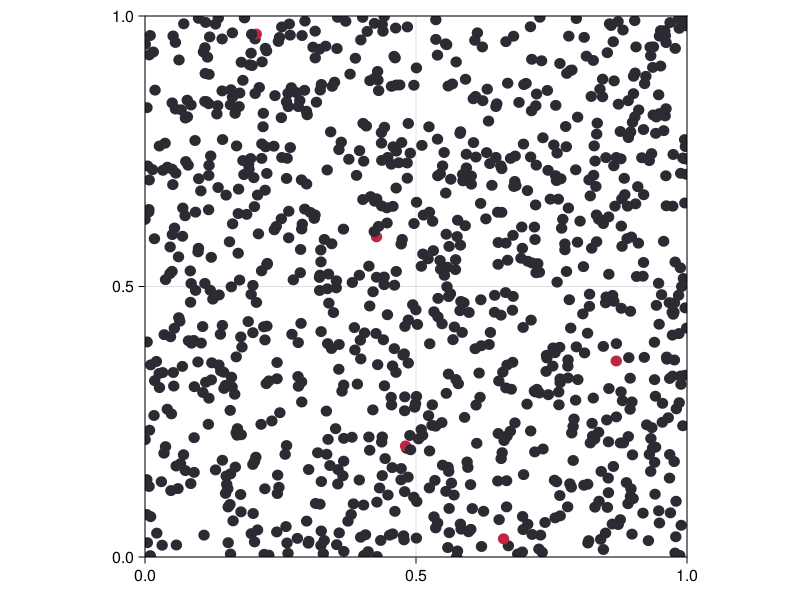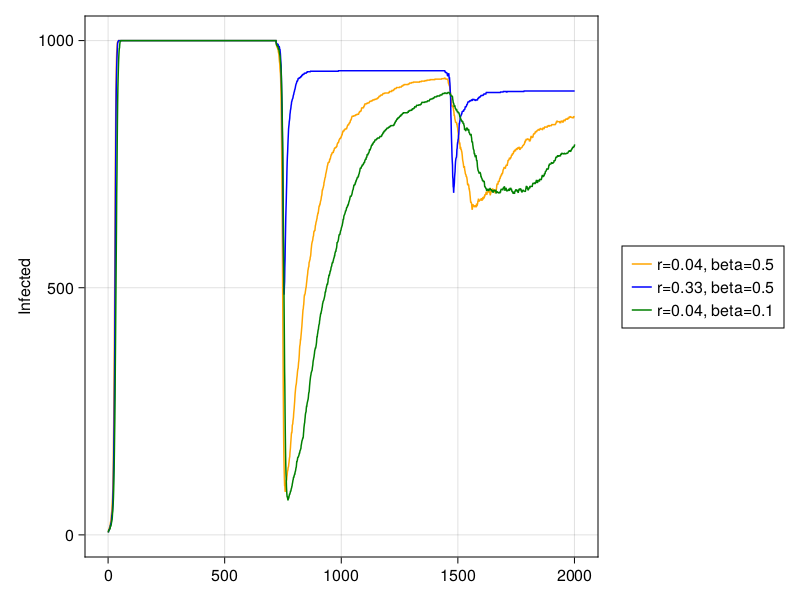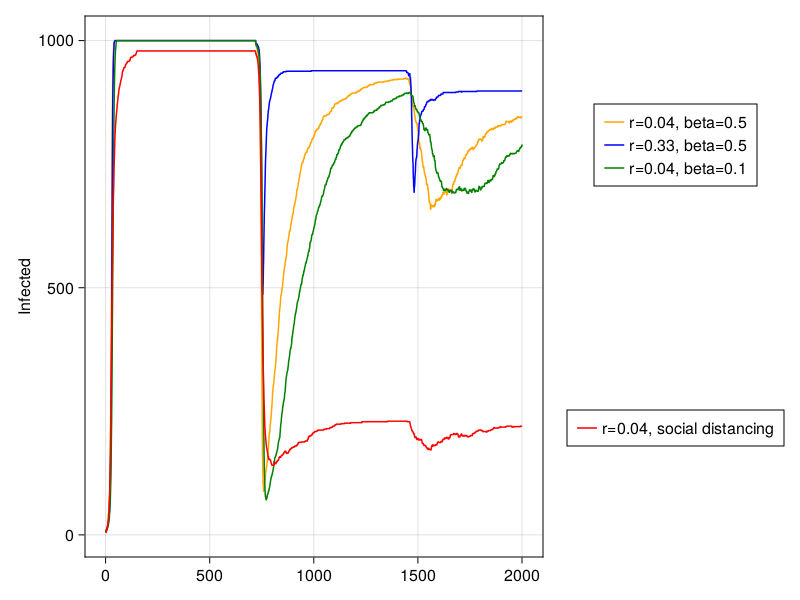# Continuous space social distancing

This is a model similar to our SIR model for the spread of COVID-19. But instead of having different cities, we let agents move in one continuous space and transfer the disease if they come into contact with one another. This model is partly inspired by this article, and can complement the SIR graph model. The graph model can model virus transfer between cities, whilst this model can be used to study what happens within a city.

The example here serves additionally as an introduction to using continuous space, modelling billiard-like collisions in that space, and animating the agent motion in the space. Notice that a detailed description of the basics of the model regarding disease spreading exists in the SIR example, and is not repeated here.

It is also available from the Models module as Models.social_distancing.

## Moving agents in continuous space

Let us first create a simple model where balls move around in a continuous space. We need to create agents that comply with ContinuousSpace, i.e. they have a pos and vel fields, both of which are tuples of float numbers.

using Agents, Random

@agent SocialAgent ContinuousAgent{2} begin
mass::Float64
end

The mass field will come in handy later on, when we implement social isolation (i.e. that some agents don't move and can't be moved).

Let's also initialize a trivial model with continuous space

function ball_model(; speed = 0.002)
space2d = ContinuousSpace((1, 1); spacing = 0.02)
model = ABM(SocialAgent, space2d, properties = Dict(:dt => 1.0), rng = MersenneTwister(42))

# And add some agents to the model
for ind in 1:500
pos = Tuple(rand(model.rng, 2))
vel = sincos(2π * rand(model.rng)) .* speed
end
return model
end

model = ball_model()
AgentBasedModel with 500 agents of type SocialAgent
space: periodic continuous space with (1.0, 1.0) extent and spacing=0.02
scheduler: fastest
properties: dt

We took advantage of the functionality of add_agent! that creates the agents automatically. For now all agents have the same absolute speed, and mass.

The agent step function for now is trivial. It is just move_agent! in continuous space

agent_step!(agent, model) = move_agent!(agent, model, model.dt)
agent_step! (generic function with 1 method)

dt is our time resolution, but we will talk about this more later!

## Billiard-like interaction

We will model the agents as balls that collide with each other. To this end, we will use two functions from the continuous space API:

We want all agents to interact in one go, and we want to avoid double interactions (as instructed by interacting_pairs), so we define a model step.

function model_step!(model)
for (a1, a2) in interacting_pairs(model, 0.012, :nearest)
elastic_collision!(a1, a2, :mass)
end
end

model2 = ball_model()
AgentBasedModel with 500 agents of type SocialAgent
space: periodic continuous space with (1.0, 1.0) extent and spacing=0.02
scheduler: fastest
properties: dt

And then make an animation

using InteractiveDynamics
using CairoMakie

abmvideo(
"socialdist2.mp4",
model2,
agent_step!,
model_step!;
title = "Billiard-like",
frames = 50,
spf = 2,
framerate = 25,
)

Alright, this works great so far!

Agents.jl is not a billiards simulator!

Please understand that Agents.jl does not accurately simulate billiard systems. This is the job of Julia packages HardSphereDynamics.jl or DynamicalBilliards.jl. In Agents.jl we only provide an approximating function elastic_collision!. The accuracy of this simulation increases as the time resolution dt decreases, but even in the limit dt → 0 we still don't reach the accuracy of proper billiard packages.

Also notice that the plotted size of the circles representing agents is not deduced from the interaction_radius (as it should). We only eye-balled it to look similar enough.

## Immovable agents

For the following social distancing example, it will become crucial that some agents don't move, and can't be moved (i.e. they stay "isolated"). This is very easy to do with the elastic_collision! function, we only have to make some agents have infinite mass

model3 = ball_model()

for id in 1:400
agent = model3[id]
agent.mass = Inf
agent.vel = (0.0, 0.0)
end

let's animate this again

abmvideo(
"socialdist3.mp4",
model3,
agent_step!,
model_step!;
title = "Billiard-like with stationary agents",
frames = 50,
spf = 2,
framerate = 25,
)

We now add more functionality to these agents, according to the SIR model (see previous example). They can be infected with a disease and transfer the disease to other agents around them.

@agent PoorSoul ContinuousAgent{2} begin
mass::Float64
days_infected::Int  # number of days since is infected
status::Symbol  # :S, :I or :R
β::Float64
end

Here β is the transmission probability, which we choose to make an agent parameter instead of a model parameter. It reflects the level of hygiene of an individual. In a realistic scenario, the actual virus transmission would depend on the β value of both agents, but we don't do that here for simplicity.

We also significantly modify the model creation, to have SIR-related parameters. Each step in the model corresponds to one hour.

const steps_per_day = 24

using DrWatson: @dict
function sir_initiation(;
infection_period = 30 * steps_per_day,
detection_time = 14 * steps_per_day,
reinfection_probability = 0.05,
isolated = 0.0, # in percentage
dt = 1.0,
speed = 0.002,
death_rate = 0.044, # from website of WHO
N = 1000,
initial_infected = 5,
seed = 42,
βmin = 0.4,
βmax = 0.8,
)

properties = (;
infection_period,
reinfection_probability,
detection_time,
death_rate,
dt,
)
space = ContinuousSpace((1,1); spacing = 0.02)
model = ABM(PoorSoul, space, properties = properties, rng = MersenneTwister(seed))

for ind in 1:N
pos = Tuple(rand(model.rng, 2))
status = ind ≤ N - initial_infected ? :S : :I
isisolated = ind ≤ isolated * N
mass = isisolated ? Inf : 1.0
vel = isisolated ? (0.0, 0.0) : sincos(2π * rand(model.rng)) .* speed

# very high transmission probability
# we are modelling close encounters after all
β = (βmax - βmin) * rand(model.rng) + βmin
add_agent!(pos, model, vel, mass, 0, status, β)
end

return model
end
sir_initiation (generic function with 1 method)

Notice the constant steps_per_day, which approximates how many model steps correspond to one day (since the parameters we used in the previous graph SIR example were given in days).

To visualize this model, we will use black color for the susceptible, red for the infected infected and green for the recovered, leveraging InteractiveDynamics.abmplot.

sir_model = sir_initiation()

sir_colors(a) = a.status == :S ? "#2b2b33" : a.status == :I ? "#bf2642" : "#338c54"

fig, ax, abmp = abmplot(sir_model; ac = sir_colors)
fig # display figureWe have increased the size of the model 10-fold (for more realistic further analysis)

To actually spread the virus, we modify the model_step! function, so that individuals have a probability to transmit the disease as they interact.

function transmit!(a1, a2, rp)
# for transmission, only 1 can have the disease (otherwise nothing happens)
count(a.status == :I for a in (a1, a2)) ≠ 1 && return
infected, healthy = a1.status == :I ? (a1, a2) : (a2, a1)

rand(model.rng) > infected.β && return

if healthy.status == :R
rand(model.rng) > rp && return
end
healthy.status = :I
end

function sir_model_step!(model)
for (a1, a2) in interacting_pairs(model, r, :nearest)
transmit!(a1, a2, model.reinfection_probability)
elastic_collision!(a1, a2, :mass)
end
end
sir_model_step! (generic function with 1 method)

Notice that it is not necessary that the transmission interaction radius is the same as the billiard-ball dynamics. We only have them the same here for convenience, but in a real model they will probably differ.

We also modify the agent_step! function, so that we keep track of how long the agent has been infected, and whether they have to die or not.

function sir_agent_step!(agent, model)
move_agent!(agent, model, model.dt)
update!(agent)
recover_or_die!(agent, model)
end

update!(agent) = agent.status == :I && (agent.days_infected += 1)

function recover_or_die!(agent, model)
if agent.days_infected ≥ model.infection_period
if rand(model.rng) ≤ model.death_rate
kill_agent!(agent, model)
else
agent.status = :R
agent.days_infected = 0
end
end
end
recover_or_die! (generic function with 1 method)

Alright, now we can animate this process for default parameters

sir_model = sir_initiation()

abmvideo(
"socialdist4.mp4",
sir_model,
sir_agent_step!,
sir_model_step!;
title = "SIR model",
frames = 50,
ac = sir_colors,
as = 10,
spf = 1,
framerate = 20,
)

We can all agree that these animations look interesting, but let's do some actual analysis of this model. The quantity we wish to look at is the number of infected over time, so let's calculate this, similarly with the graph SIR model.

infected(x) = count(i == :I for i in x)
recovered(x) = count(i == :R for i in x)
adata = [(:status, infected), (:status, recovered)]
2-element Vector{Tuple{Symbol, Function}}:
(:status, Main.infected)
(:status, Main.recovered)

Let's do the following runs, with different parameters probabilities

r1, r2 = 0.04, 0.33
β1, β2 = 0.5, 0.1
sir_model1 = sir_initiation(reinfection_probability = r1, βmin = β1)
sir_model2 = sir_initiation(reinfection_probability = r2, βmin = β1)
sir_model3 = sir_initiation(reinfection_probability = r1, βmin = β2)

data1, _ = run!(sir_model1, sir_agent_step!, sir_model_step!, 2000; adata)
data2, _ = run!(sir_model2, sir_agent_step!, sir_model_step!, 2000; adata)
data3, _ = run!(sir_model3, sir_agent_step!, sir_model_step!, 2000; adata)

data1[(end-10):end, :]
11×3 DataFrame
Rowstepinfected_statusrecovered_status
Int64Int64Int64
1199084476
2199184476
3199284476
4199384476
5199484476
6199584476
7199684674
8199784674
9199884674
10199984674
11200084674

Now, we can plot the number of infected versus time

using CairoMakie
figure = Figure()
ax = figure[1, 1] = Axis(figure; ylabel = "Infected")
l1 = lines!(ax, data1[:, dataname((:status, infected))], color = :orange)
l2 = lines!(ax, data2[:, dataname((:status, infected))], color = :blue)
l3 = lines!(ax, data3[:, dataname((:status, infected))], color = :green)
figure[1, 2][1,1] =
Legend(figure, [l1, l2, l3], ["r=$r1, beta=$β1", "r=$r2, beta=$β1", "r=$r1, beta=$β2"])
figureExponential growth is evident in all cases.

## Social distancing

Of course in reality a dampening mechanism will (hopefully) happen before all of the population is infected: a vaccine. This effectively introduces a 4th type of status, :V for vaccinated. This type can't get infected, and thus all remaining individuals that are already infected will (hopefully) survive or die out.

Until that point, social distancing is practiced. The best way to model social distancing is to make some agents simply not move (which feels like it approximates reality better).

sir_model = sir_initiation(isolated = 0.8)
abmvideo(
"socialdist5.mp4",
sir_model,
sir_agent_step!,
sir_model_step!;
title = "Social Distancing",
frames = 100,
spf = 2,
ac = sir_colors,
framerate = 20,
)

Here we let some 20% of the population not be isolated, probably teenagers still partying, or anti-vaxers / flat-earthers that don't believe in science. Still, you can see that the spread of the virus is dramatically contained.

Let's look at the actual numbers, because animations are cool, but science is even cooler.

r4 = 0.04
sir_model4 = sir_initiation(reinfection_probability = r4, βmin = β1, isolated = 0.8)

data4, _ = run!(sir_model4, sir_agent_step!, sir_model_step!, 2000; adata)

l4 = lines!(ax, data4[:, dataname((:status, infected))], color = :red)
figure[1, 2][2,1] = Legend(
figure,
[l4],
["r=\$r4, social distancing"],
)
figureHere you can see the characteristic "flattening the curve" phrase you hear all over the news.